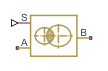# Variable Orifice Between Round Holes

Hydraulic variable orifice at intersection of two holes

## Library

Orifices

•## Description

The Variable Orifice Between Round Holes block models a variable orifice created by two interacting round holes. These holes can have different diameters. One hole is located in the sleeve, while the other is drilled in the case, with the sleeve sliding along the case. Such a configuration is frequently seen in cartridge valves, as shown in this 3-way valve schematic.The block can contain multiple identical interacting pairs of holes. The following schematic shows the calculation diagram for one such pair of round holes, where

 s Sleeve displacement from initial position c Distance between hole centers ds Sleeve hole diameter dc Case hole diameterThe flow rate through the orifice is proportional to the orifice area and to the pressure differential across the orifice, according to these equations:

`$q=z\cdot {C}_{D}\cdot A\sqrt{\frac{2}{\rho }}\cdot \frac{p}{{\left({p}^{2}+{p}_{cr}^{2}\right)}^{1/4}}$`
`$\Delta p={p}_{\text{A}}-{p}_{\text{B}},$`

where

 q Flow rate p Pressure differential pA, pB Gauge pressures at the block terminals A Instantaneous passage area between orifices c Instantaneous distance between hole centers r Smaller hole radius R Larger hole radius z Number of hole pairs CD Flow discharge coefficient ρ Fluid density Aleak Closed orifice leakage area pcr Minimum pressure for turbulent flow

The minimum pressure for turbulent flow, pcr, is calculated according to the laminar transition specification method:

• By pressure ratio — The transition from laminar to turbulent regime is defined by the following equations:

pcr = (pavg + patm)(1 – Blam)

pavg = (pA + pB)/2

where

 pavg Average pressure between the block terminals patm Atmospheric pressure, 101325 Pa Blam Pressure ratio at the transition between laminar and turbulent regimes (Laminar flow pressure ratio parameter value)
• By Reynolds number — The transition from laminar to turbulent regime is defined by the following equations:

`${p}_{cr}=\frac{\pi }{4A}{\left(\frac{{\mathrm{Re}}_{cr}}{{C}_{D}}\right)}^{2}\cdot \rho \cdot \frac{{\nu }^{2}}{2}$`

where

 ν Fluid kinematic viscosity Recr Critical Reynolds number (Critical Reynolds number parameter value)

The block positive direction is from port A to port B. This means that the flow rate is positive if it flows from A to B. Positive signal at port S moves the sleeve in the positive direction.

## Basic Assumptions and Limitations

Inertial effects are not taken into account.

## Parameters

Sleeve hole diameter

Diameter of the holes drilled in the sleeve. The default value is `0.005` m.

Case hole diameter

Diameter of the holes drilled in the case. The default value is `0.0054` m.

Number of interacting pairs

Number of interacting hole pairs. The default value is `1`.

Flow discharge coefficient

Semi-empirical parameter for orifice capacity characterization. Its value depends on the geometrical properties of the orifice, and usually is provided in textbooks or manufacturer data sheets. The default value is `0.7`.

Laminar transition specification

Select how the block transitions between the laminar and turbulent regimes:

• `Pressure ratio` — The transition from laminar to turbulent regime is smooth and depends on the value of the Laminar flow pressure ratio parameter. This method provides better simulation robustness.

• `Reynolds number` — The transition from laminar to turbulent regime is assumed to take place when the Reynolds number reaches the value specified by the Critical Reynolds number parameter.

Laminar flow pressure ratio

Pressure ratio at which the flow transitions between laminar and turbulent regimes. The default value is `0.999`. This parameter is visible only if the Laminar transition specification parameter is set to `Pressure ratio`.

Critical Reynolds number

The maximum Reynolds number for laminar flow. The value of the parameter depends on the orifice geometrical profile. You can find recommendations on the parameter value in hydraulics textbooks. The default value is `12`. This parameter is visible only if the Laminar transition specification parameter is set to `Reynolds number`.

Leakage area

The total area of possible leaks in the completely closed orifice. The main purpose of the parameter is to maintain numerical integrity of the circuit by preventing a portion of the system from getting isolated after the orifice is completely closed. The parameter value must be greater than 0. The default value is `1e-12` m^2.

## Global Parameters

Parameters determined by the type of working fluid:

• Fluid density

• Fluid kinematic viscosity

Use the Hydraulic Fluid block or the Custom Hydraulic Fluid block to specify the fluid properties.

## Ports

The block has the following ports:

`A`

Hydraulic conserving port associated with the orifice inlet.

`B`

Hydraulic conserving port associated with the orifice outlet.

`S`

Physical signal port that provides the instantaneous value of the distance between the hole centers.

## Version History

Introduced in R2014b